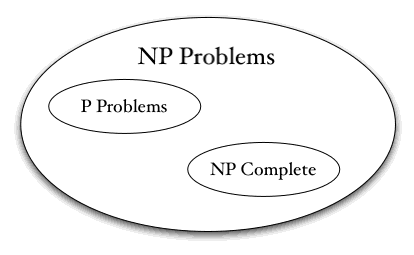Explore BrainMass
Share

Computational Complexity Theory

Computational Complexity Theory is arguably the most important aspect of the Theory of Computation. The theory seeks to assign a classification to a problem based on how inherently difficult it is to solve. The general process to determine complexity is to take the input as size n, and find how many operations proportional to n are required to solve the problem.

Complexity is most often applied to decision problems butt can be applied to other problem classes by defining what the algorithm has to do to give a desired output. There are several classes of complexity. Some deal with the time complexity, or how long the algorithm can be expected to run, while others deal with the space complexity, or how much memory the algorithm will take to do its job. The most common time complexity classes are P, NP-Complete and NP-hard, which are the polynomial classes. Other time complexity classes include EXPTIME, which is exponential polynomial time, and is the most inherently difficult class of problems. Space complexity is less discussed because memory is a comparatively cheap resource now that we can produce very large memory pools. When dealing with complexity, computer scientists use Big-O notation to denote it.The more difficult classes include the classes below them, meaning that any problem in NP is also in EXPTIME, but not every problem in EXPTIME is in NP. This means that if a problem is in NP, there exists a solution that takes NP time, but there is also a solution that takes EXPTIME time. When crafting algorithms to solve problems, the programmer will always want to find the least-time-complex algorithm possible. For many problems, it is unknown what the exact class of the problem is, and if better solutions exist.

© BrainMass Inc. brainmass.com October 23, 2019, 7:18 pm ad1c9bdddf